1. Worksheets>
2. Math>
3. Pre-Algebra>
4. Integers>
5. Multiplication and Division

Integers: Multiplication and Division Worksheets

These printable pages contain worksheets, which emphasize integer multiplication and division. Practice pages here contain exercises on multiplication squares, in-out boxes, evaluating expressions, filling in missing integers, and more.

Integer multiplication chart

These chart are prepared in such a way that students learn to multiply integers following the multiplication sign rule. Print and practice the blank charts.Display chart:

Blank chart:

Multiplication of integers

Multiply the integers to find the product. A total of 48 problems are given in these integer worksheets for practice.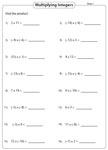Multiplication squares

In these worksheets, 2X2 and 3X3 multiplication squares are given. Multiply the integers in the rows and columns and write the products in the squares.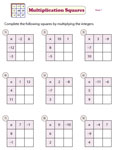2x2:

3x3:

Multiplying 3 or 4 integers

Find the product of the integers. Apply the multiplication sign rule. Each worksheet consists of ten problems.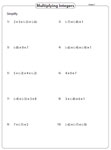Dividing integers

Perform the division operation on the integers to find the quotient in these three worksheets.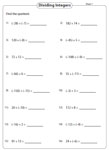Multiplying and dividing integers

Simplify the integer equations by performing multiplication and division operations.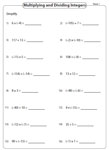Missing integers: Multiplication and division

Find the missing integer in each equation. There are 16 problems in each worksheet.In-and-out boxes: Multiply and divide

Fill in the in-and-out boxes according to the rule mentioned in each problem. There are six rules given in each worksheet.Evaluate: Multiply and divide

Evaluate the expressions by substituting the values in the variables. Each worksheet contains four problems with three expressions each.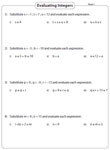Stock up your library with the complete set of worksheets on multiplying and dividing integers.